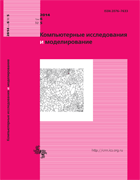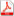Issue 5, 2014 Vol. 6

# All issues

Incomplete systems of linear equations with restrictions of variable valuespdf (657K)  / List of references

The problem is formulated for description of objects having various natures which uses a system of linear equations with variable number exceeding the number of the equations. An important feature of this problem that substantially complicates its solving is the existing of restrictions imposed on a number of the variables. In particular, the choice of biochemical reaction aggregate that converts a preset substrate (a feedstock) into a preset product belongs to this kind of problems. In this case, unknown variables are the rates of biochemical reactions which form a vector to be determined. Components of this vector are subdivided into two groups: 1) the defined components, $\vec{y}$; 2) those dependent on the defined ones, $\vec{x}$. Possible configurations of the domain of $\vec{y}$ values permitted by restrictions imposed upon $\vec{x}$ components have been studied. It has been found that a part of restrictions may be superfluous and, therefore, unnecessary for the problem solving. Situations are analyzed when two or more $\vec{x}$ restrictions result in strict interconnections between $\vec{y}$ components. Methods of search of the basis solutions which take into account the peculiarities of this problem are described. Statement of the general problem and properties of its solutions are illustrated using a biochemical example.

Keywords: linear equations, non-square matrices, linear inequalities, stoichiometry of metabolism, metabolic pathways
Citation in English: Minkevich I.G. Incomplete systems of linear equations with restrictions of variable values // Computer Research and Modeling, 2014, vol. 6, no. 5, pp. 719-745
Citation in English: Minkevich I.G. Incomplete systems of linear equations with restrictions of variable values // Computer Research and Modeling, 2014, vol. 6, no. 5, pp. 719-745
DOI: 10.20537/2076-7633-2014-6-5-719-745
• . Stoichiometric synthesis of metabolic pathways. // Computer Research and Modeling. 2015. — V. 7, no. 6. — P. 1241.
Please note that citation information may be incomplete as it includes data from Crossref cited-by program partners only.
Views (last year): 24. Citations: 3 (RSCI).

Full-text version of the journal is also available on the web site of the scientific electronic library eLIBRARY.RU

The journal is included in the Russian Science Citation Index

The journal is included in the List of Russian peer-reviewed journals publishing the main research results of PhD and doctoral dissertations.

International Interdisciplinary Conference "Mathematics. Computing. Education"

The journal is included in the RSCI

Indexed in Scopus